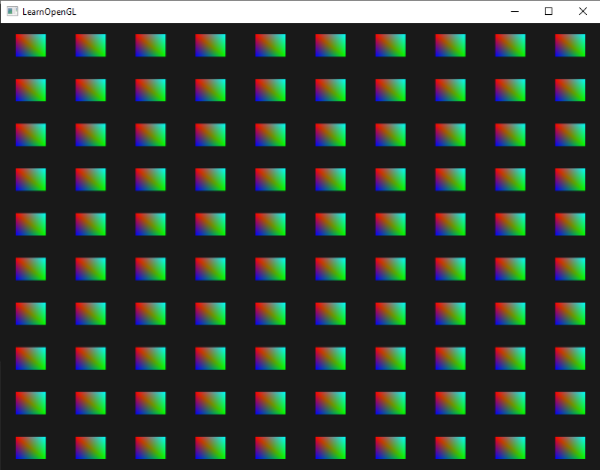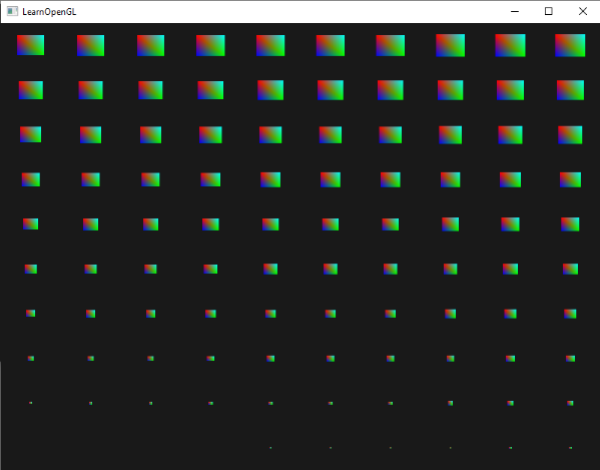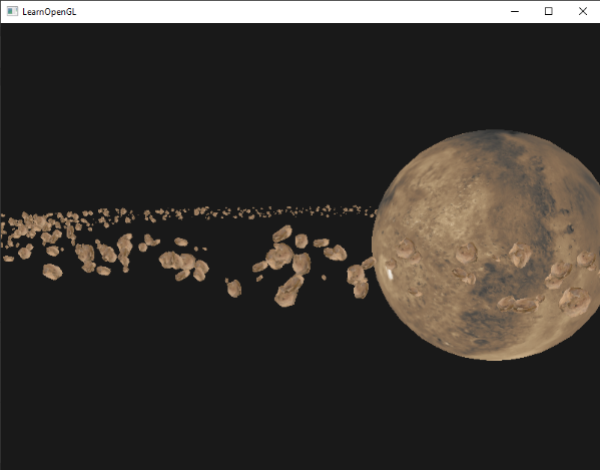# 实例化

for(GLuint i = 0; i < amount_of_models_to_draw; i++)
{
DoSomePreparations(); //在这里绑定VAO、绑定纹理、设置uniform变量等
glDrawArrays(GL_TRIANGLES, 0, amount_of_vertices);
}GLfloat quadVertices[] = {
//  ---位置---   ------颜色-------
-0.05f,  0.05f,  1.0f, 0.0f, 0.0f,
0.05f, -0.05f,  0.0f, 1.0f, 0.0f,
-0.05f, -0.05f,  0.0f, 0.0f, 1.0f,

-0.05f,  0.05f,  1.0f, 0.0f, 0.0f,
0.05f, -0.05f,  0.0f, 1.0f, 0.0f,
0.05f,  0.05f,  0.0f, 1.0f, 1.0f
};


#version 330 core
in vec3 fColor;
out vec4 color;

void main()
{
color = vec4(fColor, 1.0f);
}


#version 330 core
layout (location = 0) in vec2 position;
layout (location = 1) in vec3 color;

out vec3 fColor;

uniform vec2 offsets;

void main()
{
vec2 offset = offsets[gl_InstanceID];
gl_Position = vec4(position + offset, 0.0f, 1.0f);
fColor = color;
}


glm::vec2 translations;
int index = 0;
GLfloat offset = 0.1f;
for(GLint y = -10; y < 10; y += 2)
{
for(GLint x = -10; x < 10; x += 2)
{
glm::vec2 translation;
translation.x = (GLfloat)x / 10.0f + offset;
translation.y = (GLfloat)y / 10.0f + offset;
translations[index++] = translation;
}
}


shader.Use();
for(GLuint i = 0; i < 100; i++)
{
stringstream ss;
string index;
ss << i;
index = ss.str();
GLint location = glGetUniformLocation(shader.Program, ("offsets[" + index + "]").c_str())
glUniform2f(location, translations[i].x, translations[i].y);
}


glBindVertexArray(quadVAO);
glDrawArraysInstanced(GL_TRIANGLES, 0, 6, 100);
glBindVertexArray(0);


glDrawArraysInstanced的参数和glDrawArrays一样，除了最后一个参数设置了我们打算绘制实例的数量。我们想展示100个四边形，它们以10×10网格形式展现，所以这儿就是100.运行代码，你会得到100个相似的有色四边形。

## 实例化数组

#version 330 core
layout (location = 0) in vec2 position;
layout (location = 1) in vec3 color;
layout (location = 2) in vec2 offset;

out vec3 fColor;

void main()
{
gl_Position = vec4(position + offset, 0.0f, 1.0f);
fColor = color;
}


GLuint instanceVBO;
glGenBuffers(1, &instanceVBO);
glBindBuffer(GL_ARRAY_BUFFER, instanceVBO);
glBufferData(GL_ARRAY_BUFFER, sizeof(glm::vec2) * 100, &translations, GL_STATIC_DRAW);
glBindBuffer(GL_ARRAY_BUFFER, 0);


glEnableVertexAttribArray(2);
glBindBuffer(GL_ARRAY_BUFFER, instanceVBO);
glVertexAttribPointer(2, 2, GL_FLOAT, GL_FALSE, 2 * sizeof(GLfloat), (GLvoid*)0);
glBindBuffer(GL_ARRAY_BUFFER, 0);
glVertexAttribDivisor(2, 1);void main()
{
vec2 pos = position * (gl_InstanceID / 100.0f);
gl_Position = vec4(pos + offset, 0.0f, 1.0f);
fColor = color;
}# 小行星带

GLuint amount = 1000;
glm::mat4* modelMatrices;
modelMatrices = new glm::mat4[amount];
srand(glfwGetTime()); // initialize random seed
GLfloat offset = 2.5f;
for(GLuint i = 0; i < amount; i++)
{
glm::mat4 model;
// 1. Translation: displace along circle with 'radius' in range [-offset, offset]
GLfloat angle = (GLfloat)i / (GLfloat)amount * 360.0f;
GLfloat displacement = (rand() % (GLint)(2 * offset * 100)) / 100.0f - offset;
GLfloat x = sin(angle) * radius + displacement;
displacement = (rand() % (GLint)(2 * offset * 100)) / 100.0f - offset;
GLfloat y = displacement * 0.4f; // y value has smaller displacement
displacement = (rand() % (GLint)(2 * offset * 100)) / 100.0f - offset;
GLfloat z = cos(angle) * radius + displacement;
model = glm::translate(model, glm::vec3(x, y, z));
// 2. Scale: Scale between 0.05 and 0.25f
GLfloat scale = (rand() % 20) / 100.0f + 0.05;
model = glm::scale(model, glm::vec3(scale));
// 3. Rotation: add random rotation around a (semi)randomly picked rotation axis vector
GLfloat rotAngle = (rand() % 360);
model = glm::rotate(model, rotAngle, glm::vec3(0.4f, 0.6f, 0.8f));
// 4. Now add to list of matrices
modelMatrices[i] = model;
}


// 绘制行星
glm::mat4 model;
model = glm::translate(model, glm::vec3(0.0f, -5.0f, 0.0f));
model = glm::scale(model, glm::vec3(4.0f, 4.0f, 4.0f));
glUniformMatrix4fv(modelLoc, 1, GL_FALSE, glm::value_ptr(model));

// 绘制石头
for(GLuint i = 0; i < amount; i++)
{
glUniformMatrix4fv(modelLoc, 1, GL_FALSE, glm::value_ptr(modelMatrices[i]));
}#version 330 core
layout (location = 0) in vec3 position;
layout (location = 2) in vec2 texCoords;
layout (location = 3) in mat4 instanceMatrix;

out vec2 TexCoords;

uniform mat4 projection;
uniform mat4 view;

void main()
{
gl_Position = projection * view * instanceMatrix * vec4(position, 1.0f);
TexCoords = texCoords;
}


for(GLuint i = 0; i < rock.meshes.size(); i++)
{
GLuint VAO = rock.meshes[i].VAO;
// Vertex Buffer Object
GLuint buffer;
glBindVertexArray(VAO);
glGenBuffers(1, &buffer);
glBindBuffer(GL_ARRAY_BUFFER, buffer);
glBufferData(GL_ARRAY_BUFFER, amount * sizeof(glm::mat4), &modelMatrices, GL_STATIC_DRAW);
// Vertex Attributes
GLsizei vec4Size = sizeof(glm::vec4);
glEnableVertexAttribArray(3);
glVertexAttribPointer(3, 4, GL_FLOAT, GL_FALSE, 4 * vec4Size, (GLvoid*)0);
glEnableVertexAttribArray(4);
glVertexAttribPointer(4, 4, GL_FLOAT, GL_FALSE, 4 * vec4Size, (GLvoid*)(vec4Size));
glEnableVertexAttribArray(5);
glVertexAttribPointer(5, 4, GL_FLOAT, GL_FALSE, 4 * vec4Size, (GLvoid*)(2 * vec4Size));
glEnableVertexAttribArray(6);
glVertexAttribPointer(6, 4, GL_FLOAT, GL_FALSE, 4 * vec4Size, (GLvoid*)(3 * vec4Size));

glVertexAttribDivisor(3, 1);
glVertexAttribDivisor(4, 1);
glVertexAttribDivisor(5, 1);
glVertexAttribDivisor(6, 1);

glBindVertexArray(0);
}


// Draw meteorites
for(GLuint i = 0; i < rock.meshes.size(); i++)
{
glBindVertexArray(rock.meshes[i].VAO);
glDrawElementsInstanced(
GL_TRIANGLES, rock.meshes[i].vertices.size(), GL_UNSIGNED_INT, 0, amount
);
glBindVertexArray(0);
}Important